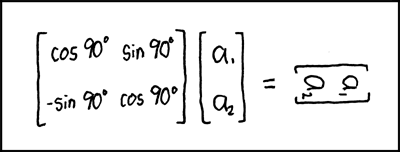Classes of Abed Nego Ragil Putra > PHP Inventory Management System with Scanner > vendor/markbaker/matrix/README.md > Download
File: vendor/markbaker/matrix/README.md Documentation text/markdown Documentation PHP Inventory Management System with Scanner Manage inventory stock using scanner application By Abed Nego Ragil Putra 2 years ago 3,990 bytes

## ContentsDownload

# PHPMatrix

PHP Class for handling MatricesMatrix Transform

This library currently provides the following operations:

- addition - direct sum - subtraction - multiplication - division (using [A].[B]<sup>-1</sup>)

``````- division by
- division into
``````

together with functions for

- adjoint - antidiagonal - cofactors - determinant - diagonal - identity - inverse - minors - trace - transpose

## TO DO

- power() - EigenValues - EigenVectors - Decomposition

# Usage

To create a new Matrix object, provide an array as the constructor argument

``````\$grid = [
[16,  3,  2, 13],
[ 5, 10, 11,  8],
[ 9,  6,  7, 12],
[ 4, 15, 14,  1],
];

\$matrix = new Matrix\Matrix(\$grid);
``````

The `Builder` class provides helper methods for creating specific matrices, specifically an identity matrix of a specified size; or a matrix of a specified dimensions, with every cell containing a set value.

``````\$matrix = new Matrix\Builder::createFilledMatrix(1, 5, 3);
``````

Will create a matrix of 5 rows and 3 columns, filled with a `1` in every cell; while

``````\$matrix = new Matrix\Builder::createIdentityMatrix(3);
``````

will create a 3x3 identity matrix.

Matrix objects are immutable: whenever you call a method or pass a grid to a function that returns a matrix value, a new Matrix object will be returned, and the original will remain unchanged. This also allows you to chain multiple methods as you would for a fluent interface (as long as they are methods that will return a Matrix result).

## Performing Mathematical Operations

To perform mathematical operations with Matrices, you can call the appropriate method against a matrix value, passing other values as arguments

``````\$matrix1 = new Matrix([
[2, 7, 6],
[9, 5, 1],
[4, 3, 8],
]);
\$matrix2 = new Matrix([
[1, 2, 3],
[4, 5, 6],
[7, 8, 9],
]);

echo \$matrix1->multiply(\$matrix2);
``````

or pass all values to the appropriate function

``````\$matrix1 = new Matrix([
[2, 7, 6],
[9, 5, 1],
[4, 3, 8],
]);
\$matrix2 = new Matrix([
[1, 2, 3],
[4, 5, 6],
[7, 8, 9],
]);

echo Matrix\multiply(\$matrix1, \$matrix2);
``````

You can pass in the arguments as Matrix objects, or as arrays.

If you want to perform the same operation against multiple values (e.g. to add three or more matrices), then you can pass multiple arguments to any of the operations.

## Using functions

When calling any of the available functions for a matrix value, you can either call the relevant method for the Matrix object

``````\$grid = [
[16,  3,  2, 13],
[ 5, 10, 11,  8],
[ 9,  6,  7, 12],
[ 4, 15, 14,  1],
];

\$matrix = new Matrix\Matrix(\$grid);

echo \$matrix->trace();
``````

or you can call the function as you would in procedural code, passing the Matrix object as an argument

``````\$grid = [
[16,  3,  2, 13],
[ 5, 10, 11,  8],
[ 9,  6,  7, 12],
[ 4, 15, 14,  1],
];

\$matrix = new Matrix\Matrix(\$grid);
echo Matrix\trace(\$matrix);
``````

When called procedurally using the function, you can pass in the argument as a Matrix object, or as an array.

``````\$grid = [
[16,  3,  2, 13],
[ 5, 10, 11,  8],
[ 9,  6,  7, 12],
[ 4, 15, 14,  1],
];

echo Matrix\trace(\$grid);
``````

As an alternative, it is also possible to call the method directly from the `Functions` class.

``````\$grid = [
[16,  3,  2, 13],
[ 5, 10, 11,  8],
[ 9,  6,  7, 12],
[ 4, 15, 14,  1],
];

\$matrix = new Matrix\Matrix(\$grid);
echo Matrix\Functions::trace(\$matrix);
``````

Used this way, methods must be called statically, and the argument must be the Matrix object, and cannot be an array.Advertise on this siteSite mapNewsletterStatisticsSite tipsPrivacy policyContact
For more information send a message to `info at phpclasses dot org`.